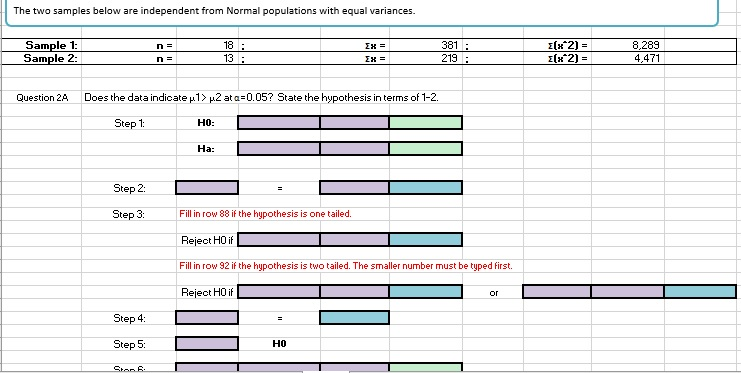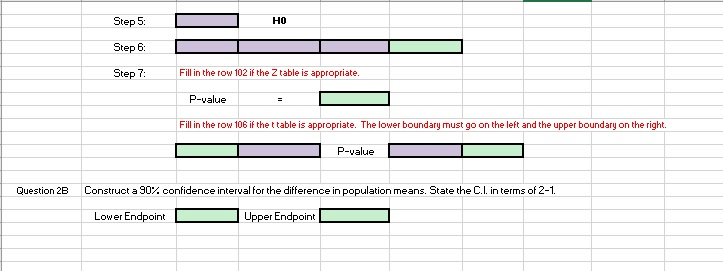# The two samples below are independent from Normal populations with equal variances. Sample 1: Sam...The two samples below are independent from Normal populations with equal variances. Sample 1: Sample 2 18 13 381 8,289 4,471 t(x^2)= Question 2A Does the data indicate μ12μ2 at α-0.05?State the hypothesis in terms of 1-2. Step t H0: Ha: Step 2: Step 3 Fill in row 88 if the hypothesis is one tailed. Reject H0 i Fill in row 92 if the hypothesis is two tailed. The smaller number must be typed first. Reject H0 if Step 4 Step 5: HO
HO Step 6: Step 7: Fill in the row 102 if the Z table is appropriate. P-value Fill in the row 106 if the ttable is appropriate. The lower boundary must go on the left and the upper boundary on the right. P-value Question 2B Construct a 90% confidence interval for the difference in population means. State the CL interms of 2-1 Lower Endpoint Upper Endpoint

2a.
Given that,
mean(x)=381
standard deviation , s.d1=91.043
number(n1)=18
y(mean)=219
standard deviation, s.d2 =66.865
number(n2)=13
null, Ho: u1 = u2
alternate, H1: u1 > u2
level of significance, α = 0.05
from standard normal table,right tailed t α/2 =1.782
since our test is right-tailed
reject Ho, if to > 1.782
we use test statistic (t) = (x-y)/sqrt(s.d1^2/n1)+(s.d2^2/n2)
to =381-219/sqrt((8288.82785/18)+(4470.92823/13))
to =5.712
| to | =5.712
critical value
the value of |t α| with min (n1-1, n2-1) i.e 12 d.f is 1.782
we got |to| = 5.71185 & | t α | = 1.782
make decision
hence value of | to | > | t α| and here we reject Ho
p-value:right tail - Ha : ( p > 5.7119 ) = 0.00005
hence value of p0.05 > 0.00005,here we reject Ho
---------------
null, Ho: u1 = u2
alternate, H1: u1 > u2
test statistic: 5.712
critical value: 1.782
decision: reject Ho
p-value: 0.00005
we have enough evidence to support the claim that sample group1 is greater than sample group2

2b.
given that,
mean(x)=381
standard deviation , s.d1=91.043
number(n1)=18
y(mean)=219
standard deviation, s.d2 =66.865
number(n2)=13
I.
standard error = sqrt(s.d1^2/n1)+(s.d2^2/n2)
where,
sd1, sd2 = standard deviation of both
n1, n2 = sample size
standard error = sqrt((8288.828/18)+(4470.928/13))
= 28.362
II.
margin of error = t a/2 * (standard error)
where,
t a/2 = t -table value
level of significance, α = 0.1
from standard normal table, two tailed and
value of |t α| with min (n1-1, n2-1) i.e 12 d.f is 1.782
margin of error = 1.782 * 28.362
= 50.541
III.
CI = (x1-x2) ± margin of error
confidence interval = [ (381-219) ± 50.541 ]
= [111.459 , 212.541]
-----------------------------------------------------------------------------------------------
DIRECT METHOD
given that,
mean(x)=381
standard deviation , s.d1=91.043
sample size, n1=18
y(mean)=219
standard deviation, s.d2 =66.865
sample size,n2 =13
CI = x1 - x2 ± t a/2 * Sqrt ( sd1 ^2 / n1 + sd2 ^2 /n2 )
where,
x1,x2 = mean of populations
sd1,sd2 = standard deviations
n1,n2 = size of both
a = 1 - (confidence Level/100)
ta/2 = t-table value
CI = confidence interval
CI = [( 381-219) ± t a/2 * sqrt((8288.828/18)+(4470.928/13)]
= [ (162) ± t a/2 * 28.362]
= [111.459 , 212.541]
-----------------------------------------------------------------------------------------------
interpretations:
1. we are 90% sure that the interval [111.459 , 212.541] contains the true population proportion
2. If a large number of samples are collected, and a confidence interval is created
for each sample, 90% of these intervals will contains the true population proportion

##### Add Answer of: The two samples below are independent from Normal populations with equal variances. Sample 1: Sam...
More Homework Help Questions Additional questions in this topic.

• #### The following data were drawn from two independent populations. Sample 1 Sample 2 15 23 19 3430 2...

Need Online Homework Help?